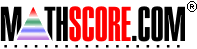Math Practice Online > free > lessons > Arizona > 2nd grade > Function Tables

These sample problems below for Function Tables were generated by the MathScore.com engine.

## Sample Problems For Function Tables

### Complexity=1, Mode=givenRule

Use the given rule to fill in the missing values.
1.
Subtract 2
Input (x)Output (y)
7
12
6
9
2.
Input (x)Output (y)
5
7
6
8

### Complexity=2, Mode=givenRule

Use the given rule to fill in the missing values.
1.
Divide by 4
Input (x)Output (y)
28
32
16
36
2.
y = x ÷ 2
Input (x)Output (y)
2
20
14
10

### Complexity=1, Mode=findRule

Find the rule that applies to the table.
1.
Rule: y = x
Input (x)Output (y)
92
103
125
169
1710
2.
Rule: y = x
Input (x)Output (y)
42
53
64
75
108

### Complexity=2, Mode=findRule

Find the rule that applies to the table.
1.
Rule: y = x
Input (x)Output (y)
153
204
255
408
459
2.
Rule: y = x
Input (x)Output (y)
17
428
642
749
963

### Complexity=1, Mode=givenRule

Use the given rule to fill in the missing values.
1
Subtract 2
Input (x)Output (y)
7
12
6
9
Solution
Subtract means "minus" or "-".
So, you get y from x minus 2.

Substitute numbers in for known x values.
x y = x - 2 y
7   y = x - 2   =   7 - 2   =   5   5
12   y = x - 2   =   12 - 2   =   10   10
6   y = x - 2   =   6 - 2   =   4   4
9   y = x - 2   =   9 - 2   =   7   7

2
Input (x)Output (y)
5
7
6
8
Solution
Add means "plus" or "+".
So, you get y from x plus 3.

Substitute numbers in for known x values.
x y = x + 3 y
5   y = x + 3   =   5 + 3   =   8   8
7   y = x + 3   =   7 + 3   =   10   10
6   y = x + 3   =   6 + 3   =   9   9
8   y = x + 3   =   8 + 3   =   11   11

### Complexity=2, Mode=givenRule

Use the given rule to fill in the missing values.
1
Divide by 4
Input (x)Output (y)
28
32
16
36
Solution
Divide means "÷".
So, you get y from x ÷ 4.

Substitute numbers in for known x values.
x y = x ÷ 4 y
28   y = x ÷ 4   =   28 ÷ 4   =   7   7
32   y = x ÷ 4   =   32 ÷ 4   =   8   8
16   y = x ÷ 4   =   16 ÷ 4   =   4   4
36   y = x ÷ 4   =   36 ÷ 4   =   9   9

2
y = x ÷ 2
Input (x)Output (y)
2
20
14
10
Solution

Substitute numbers in for known x values.
x y = x ÷ 2 y
2   y = x ÷ 2   =   2 ÷ 2   =   1   1
20   y = x ÷ 2   =   20 ÷ 2   =   10   10
14   y = x ÷ 2   =   14 ÷ 2   =   7   7
10   y = x ÷ 2   =   10 ÷ 2   =   5   5

### Complexity=1, Mode=findRule

Find the rule that applies to the table.
1
Rule: y = x
Input (x)Output (y)
92
103
125
169
1710
Solution
To find the rule, look at the given input-output pairs.
9 → 2
10 → 3
12 → 5
16 → 9
17 → 10

Each output is less than the input.
Try a rule with subtraction or division.

Since the difference between all the given pairs are different, the rule must be subtraction.

2
Rule: y = x
Input (x)Output (y)
42
53
64
75
108
Solution
To find the rule, look at the given input-output pairs.
4 → 2
5 → 3
6 → 4
7 → 5
10 → 8

Each output is less than the input.
Try a rule with subtraction or division.

Since the difference between all the given pairs are different, the rule must be subtraction.

### Complexity=2, Mode=findRule

Find the rule that applies to the table.
1
Rule: y = x
Input (x)Output (y)
153
204
255
408
459
Solution
To find the rule, look at the given input-output pairs.
15 → 3
20 → 4
25 → 5
40 → 8
45 → 9

Each output is less than the input.
Try a rule with subtraction or division.

Since the difference between all the given pairs are different, the rule must be subtraction.

2
Rule: y = x
Input (x)Output (y)
17
428
642
749
963
Solution
To find the rule, look at the given input-output pairs.
1 → 7
4 → 28
6 → 42
7 → 49
9 → 63

Each output is less than the input.
Try a rule with subtraction or division.

Since the difference between all the given pairs are different, the rule must be subtraction.

MathScore.com

Copyright Accurate Learning Systems Corporation 2008.
MathScore is a registered trademark.